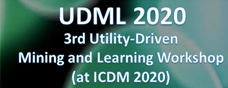The Data Mining ForumThis forum is about data mining, data science and big data: algorithms, source code, datasets, implementations, optimizations, etc. You are welcome to post call for papers, data mining job ads, link to source code of data mining algorithms or anything else related to data mining. The forum is hosted by P. Fournier-Viger. No registration is required to use this forum!.
Lexicographical order in Efim-Closed algorithm
Posted by: solmaz
Date: February 06, 2020 11:01PM

Hello, Prof. Philippe Fournier-Viger.

Sorting Transactions for Transaction Merging (HTM), the Lexicographical order of transactions is used.
How does this sort work? What is the sorting criterion?
Can you give an example?

Re: Lexicographical order in Efim-Closed algorithm
Date: February 07, 2020 12:33AM

Hi,

Yes sure.

Assume that we have a total order on items such as A,B,C,D,E ... which is the alphabetical order.

To be able to apply transaction merging efficiently, we need to sort the transactions. For some detailed example, consider the database on page 13 of this powerpoint presentation about EFIM:
http://www.philippe-fournier-viger.com/EFIM_and_EFIM-Closed_high_utility_mining.pdf

T1 {A,B,C,D,E,F}
T2 {A,B,C,D,E,F}
T3 {C,D,E, F}
T4 {D, A, G}
T5 {B, C, A, G}
T6 {A, E, F}
T7 {B, C, F, G}
T8 {A, C, D, F, G}

This database above contains 8 transactions but it is not sorted. Now we will sort the transactions in that database so that we can now do transaction merging.

We sort the transactions such that they are in alphabetical order when we read them backward. The result is the database on the right of page 13.

T6 {A, E, F}
T3 {C,D,E, F}
T1 {A,B,C,D,E,F}
T2 {A,B,C,D,E,F}
T5 {B, C, A, G}
T4 {D, A, G}
T7 {B, C, F, G}
T8 {A, C, D, F, G}

As you can see T6, T3, T1, T2 are put before T5 T4 T7 and T8 because T6, T3, T2 and T1 end with "F", while T5 T4 t7 and T8 end by "G" and "F" is before "G" according to the alphabetical order. This is illustrated here:

T6 {A, E, F}
T3 {C,D,E, F}
T1 {A,B,C,D,E,F}
T2 {A,B,C,D,E,F}
T5 {B, C, A, G}
T4 {D, A, G}
T7 {B, C, F, G}
T8 {A, C, D, F, G}

Then you can observe that T6 is put before T3 because although both of them end by E,F, the transaction T6 has a A, which is smaller than D according to the alphabetical order.

T6 {A, E, F}
T3 {C,D,E, F}

etc.

So basically, the idea of this sort is not complicated. You can think that this is like in a dictionary. In an English dictionary, the words are sorted in alphabetical order by reading the words from left to right. Here, the only difference is that we sort by alphabetical order when reading the words from right to left!

We we sort by reading the words from right to left? The reason is that by doing this we ensure that all the transactions that have the same ending will always be one after the other in the database. Thus, we can merge identical transactions by just comparing any transactions with only the transaction before and after. For example, if you look at page 15 of the powerpoint presentation, we have a database that we have projected by item "a". By doing this we have removed items to the left of transactions. But still, all transactions that have the same ending always remain one beside the other. For example, transactions

T1 {A,B,C,D,E,F}
T2 {A,B,C,D,E,F}

have the same ending (BCDEF) and are one beside the other.

By the way, I should say that in the code, we actually dont use the alphabetical order for items but the ascending order of TWU values because it is better for reducing the search space in high utility itemset mining. But above, I have use the alphabetical order just to make it easier to explain.

Hope this is clear.

Best regards